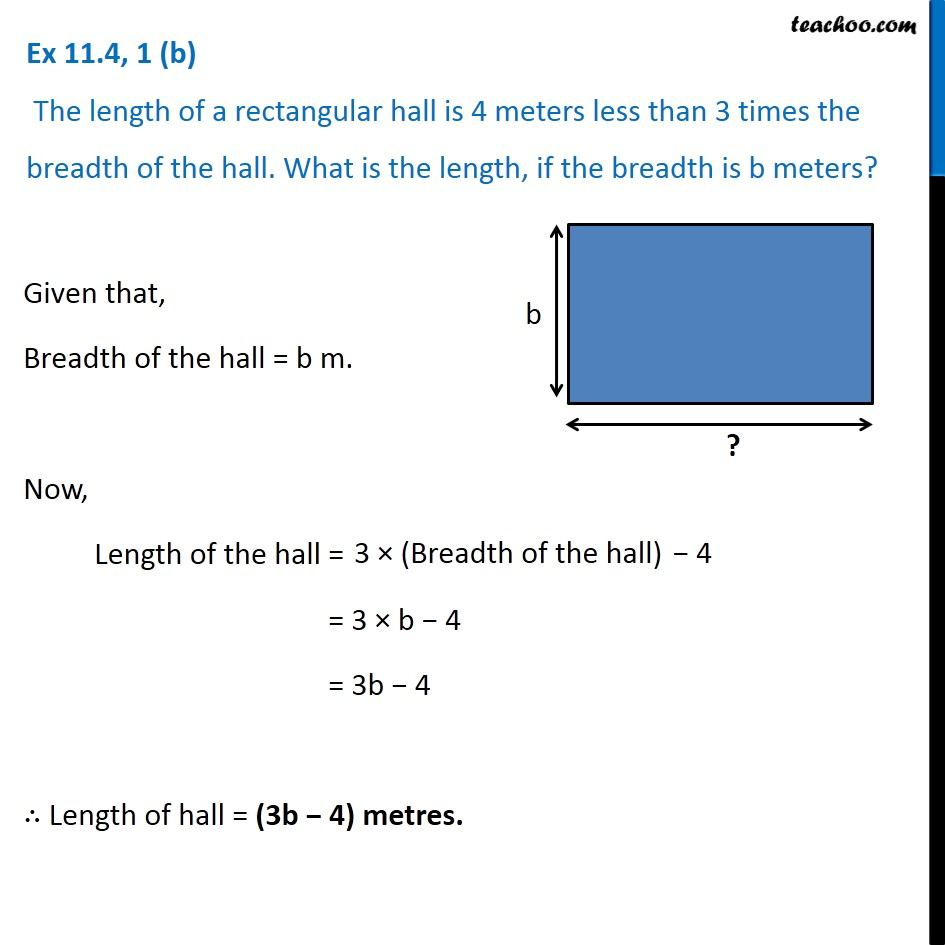Creating Algebra Expressions from Word Problems - Worksheet

Chapter 11 Class 6 Algebra
Serial order wiseLearn in your speed, with individual attention - Teachoo Maths 1-on-1 Class

### Transcript

Question 1 (b) The length of a rectangular hall is 4 meters less than 3 times the breadth of the hall. What is the length, if the breadth is b meters? Given that, Breadth of the hall = b m. Now, Length of the hall = = 3 × b − 4 = 3b − 4 ∴ Length of hall = (3b − 4) metres.